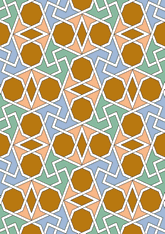data192/TUR1012

The angle of the swastika is shown as 90 degrees here. Another angle is shown in Susuz Han, Isparta-Antalya road, which is more faithful to the original.

## Geometry

• The symmetry group of the tiling is 4*2 (p4g).
• All the internal angles of the constituent polygons are a multiple of 45°.
• Contains one regular two-pointed star polygon with vertex angle of 45°.
• Contains one regular octagon.
• There is one non-regular reflective tile and one reflective pair.
• The tiling satisfies the interlace condition and has no finite interlace and two infinite interlaces with straight cross-overs.
• The tiling is edge-to-edge.
• As drawn, contains about 217 polygons.

## References

Publications referenced: